SSAT Upper Level Math : How to find the properties of an exponent

Example Questions

Example Question #80 : Expressions & Equations

What is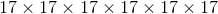in exponential notation?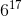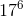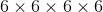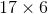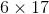Explanation:

Exponential notation includes a base number and an exponent. The base number is the number that is being multiplied and the exponent is how many times the base number is being multiplied to itself.

In this case,is our base number and it's being multiplied to itselftimes, so that is our exponent.

Example Question #81 : Expressions & Equations

What is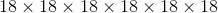in exponential notation?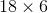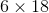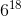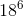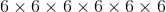Explanation:

Exponential notation includes a base number and an exponent. The base number is the number that is being multiplied and the exponent is how many times the base number is being multiplied to itself.

In this case,is our base number and it's being multiplied to itselftimes, so that is our exponent.

Example Question #82 : Expressions & Equations

What is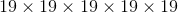in exponential notation?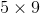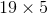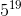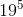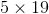Explanation:

Exponential notation includes a base number and an exponent. The base number is the number that is being multiplied and the exponent is how many times the base number is being multiplied to itself.

In this case,is our base number and it's being multiplied to itselftimes, so that is our exponent.

Example Question #83 : Expressions & Equations

What is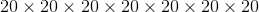in exponential notation?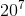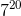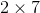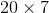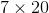Explanation:

Exponential notation includes a base number and an exponent. The base number is the number that is being multiplied and the exponent is how many times the base number is being multiplied to itself.

In this case,is our base number and it's being multiplied to itselftimes, so that is our exponent.

Example Question #84 : Expressions & Equations

What is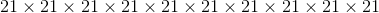in exponential notation?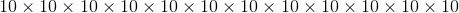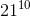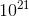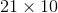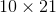Explanation:

Exponential notation includes a base number and an exponent. The base number is the number that is being multiplied and the exponent is how many times the base number is being multiplied to itself.

In this case,is our base number and it's being multiplied to itselftimes, so that is our exponent.

Example Question #85 : Expressions & Equations

What is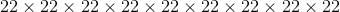in exponential notation?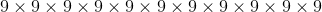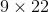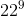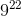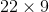Explanation:

Exponential notation includes a base number and an exponent. The base number is the number that is being multiplied and the exponent is how many times the base number is being multiplied to itself.

In this case,is our base number and it's being multiplied to itselftimes, so that is our exponent.

Example Question #86 : Expressions & Equations

What is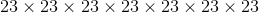in exponential notation?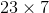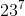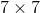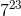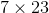Explanation:

Exponential notation includes a base number and an exponent. The base number is the number that is being multiplied and the exponent is how many times the base number is being multiplied to itself.

In this case,is our base number and it's being multiplied to itselftimes, so that is our exponent.

Example Question #87 : Expressions & Equations

What is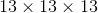in exponential notation?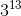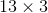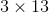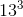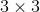Explanation:

Exponential notation includes a base number and an exponent. The base number is the number that is being multiplied and the exponent is how many times the base number is being multiplied to itself.

In this case,is our base number and it's being multiplied to itselftimes, so that is our exponent.

Example Question #81 : Expressions & Equations

What is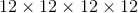in exponential notation?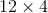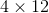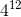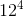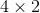Explanation:

Exponential notation includes a base number and an exponent. The base number is the number that is being multiplied and the exponent is how many times the base number is being multiplied to itself.

In this case,is our base number and it's being multiplied to itselftimes, so that is our exponent.

Example Question #89 : Expressions & Equations

What is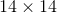in exponential notation?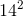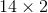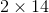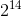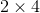In this case,is our base number and it's being multiplied to itselftimes, so that is our exponent.